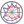Course title
 shimada akiraCourse content
This lecture gives advancesd mathematical introduction to robotics. Robotic engineering consistes of basic theory and practice. The basic theory begins with mathematical definition related to position, orientation, and coordinate frames. Furthermore, they are led to robot's rotation, transformation, and control technologies. Meanwhile, the practics includes mechanism, motor drives, electrical hardware, and software design.
They assist mutually and the both are indispensable.
The former theory has been evolved and changed gradually. Therefore, if students who want to master advanced robotics, they need to learn the advanced theory.
Purpose of class
To understand the basic theory related to advanced robotics
Goals and objectives
1. Can definite configuration spaces and the corresponding coordinate frames for robots
2. Can make equation of motions of robot manipulator with some constraints
3. Can derive jacobian-matrix for robot control
Language
English
Class schedule

Class schedule HW assignments (Including preparation and review of the class.) Amount of Time Required
1． Guidance: What's robotics? Submit the MATLAB program related to the problem. 180minutes
2． Position and Orientation Submit the MATLAB program related to the problem. 180minutes
3． Rigid body transformation Submit the MATLAB program related to the problem. 180minutes
4． Parameterization of Robotics Submit the MATLAB program related to the problem. 180minutes
5． Forward Kinematics Submit the MATLAB program related to the problem. 180minutes
6． Inverase Kinematics Submit the MATLAB program related to the problem. 180minutes
7． Velocity Kinematics Submit the MATLAB program related to the problem. 180minutes
8． Jacobian matrix Submit the MATLAB program related to the problem. 180minutes
9． Path and Trajectory Submit the MATLAB program related to the problem. 180minutes
10． Manipulability Submit the MATLAB program related to the problem. 180minutes
11． Configuration Space Submit the MATLAB program related to the problem. 190minutes
12． Planning Submit the MATLAB program related to the problem. 240minutes
13． Dynamics Submit the MATLAB program related to the problem. 240minutes
14． Control Submit the MATLAB program related to the problem. 240minutes
Total. - - 2710minutes
Relationship between 'Goals and Objectives' and 'Course Outcomes'

Problems Total.
1. 30% 30%
2. 30% 30%
3. 40% 40%
Total. 100% -
Evaluation method and criteria
Students will be evaluated based on progress reports and piriodic examination.
Textbooks and reference materials
MarkW.Spong, Seth Huchinson,M.Vidyasagar:Robot Modeling and Control,John Wiley & Sons,Inc.
Prerequisites
The students who want to master robotics should skim through the text book as a preparation. There exists a free site where we can read the all of contents.
Office hours and How to contact professors for questions